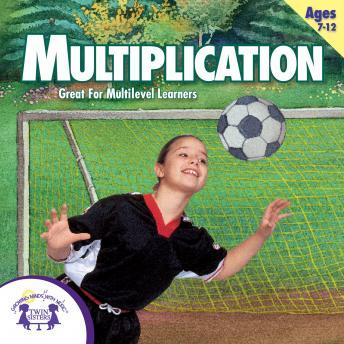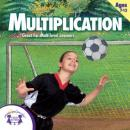# Multiplication

#### Summary:

Original songs that teach each multiplication fact 0 - 12 separately-slowly for beginners then faster for advanced learners! Then, review the facts-first with the answers and then without the answers. This album is great for the classroom or for home, and you will see immediate improvement in your child's multiplication skills! This album has over an hour of multiplication exercises that are perfect for learning and practice.
Track List:
1. You Need A Strategy
2. The Zero Property
3. It's Always The Same Number
4. The Order Doesn't Matter
5. The Skip Counting Song
6. You Can Multiply
7. Multiplication Facts Of 2
8. Multiplication Facts Of 3
9. Multiplication Facts Of 4
10. Multiplication Facts Of 5
11. Multiplication Facts Of 6
12. Multiplication Facts Of 7
13. Multiplication Facts Of 8
14. Multiplication Facts Of 9
15. Multiplication Facts of 10
16. Multiplication Facts Of 11
17. Multiplication Facts Of 12
18. Multiplication Rap For The Expert (with answers)
19. Multiplication Rap For The Expert (without answers)
Runtime: 65:32:00

## Multiplication

#### by Twin Sisters Productions

has successfully been added to your shopping cart